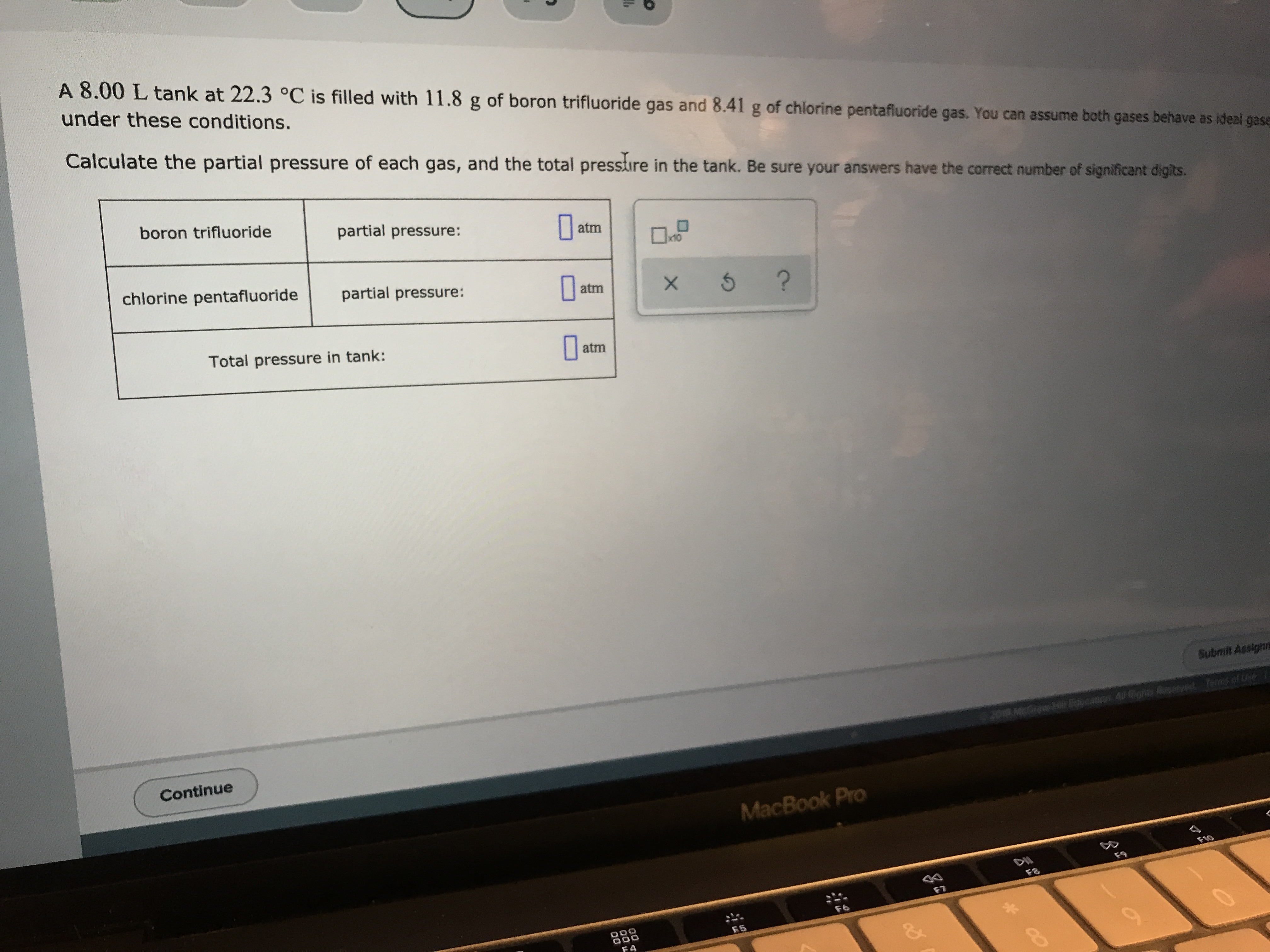# A 8.00 L tank at 22.3 oC is filled with 11.8 g of boron trifluoride gas and 8.41 g of chlorine pentafluoride gas. You can assume both gases behave as ideal gaseunder these conditions.Calculate the partial pressure of each gas, and the total presstire in the tank. Be sure your answers have the correct number of significant digits.boron trifluoridepartial pressure:atmchlorine pentafluoridepartial pressure:?XatmTotal pressure in tank:atmSubmilt AssignneContinueMacBook ProF10DWF7F6O00O00FA

Questionhelp_outlineImage TranscriptioncloseA 8.00 L tank at 22.3 oC is filled with 11.8 g of boron trifluoride gas and 8.41 g of chlorine pentafluoride gas. You can assume both gases behave as ideal gase under these conditions. Calculate the partial pressure of each gas, and the total presstire in the tank. Be sure your answers have the correct number of significant digits. boron trifluoride partial pressure: atm chlorine pentafluoride partial pressure: ? X atm Total pressure in tank: atm Submilt Assignne Continue MacBook Pro F10 DW F7 F6 O00 O00 FA fullscreen
check_circleExpert Solution
Step 1

The number of moles of BF3 (n1) is calculated using equation (1) in which m is the mass of gas, n is the number of moles, and M is the molar mass of gas.

Step 2

The number of moles of ClF5 (n2) is calculated using equation (1) as follows:

Step 3

The total number of moles of gas (nT) is calc...

### Want to see the full answer?

See Solution

#### Want to see this answer and more?

Solutions are written by subject experts who are available 24/7. Questions are typically answered within 1 hour*

See Solution
*Response times may vary by subject and question
Tagged in

### Gas laws